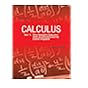Normal view MARC view ISBD view

# Calculus/ Vol. II [by] Tom M. Apostol. multi-variable calculus and linear algebra, with applications to differential equations and probability.

Material type:TextPublisher: New Delhi. Wiley India Pvt, Ltd. 2016Edition: 2d ed.Description: xxi, 673 pages; illus. 27 cm.ISBN: 9788126515202 (pbk.).Subject(s): Mathematical analysis | CalculusDDC classification: 517
Contents:
v. 1. One-variable calculus, with an introduction to linear algebra.--v. 2. Multi-variable calculus and linear algebra, with applications to differential equations and probability.
List(s) this item appears in: 2017-04-28
Item type Current location Call number Status Date due Barcode Item holds
Book Chennai Mathematical Institute
General Stacks
515 APO (Browse shelf) Available 10109
Book Chennai Mathematical Institute
General Stacks
515 APO (Browse shelf) Available 10110
Book Chennai Mathematical Institute
General Stacks
515 APO (Browse shelf) Available 10111
Book Chennai Mathematical Institute
General Stacks
515 APO (Browse shelf) Available 10112
Book Chennai Mathematical Institute
General Stacks
515 APO (Browse shelf) Available 10113
Total holds: 0
##### Browsing Chennai Mathematical Institute Shelves , Shelving location: General Stacks Close shelf browser515 APO Calculus / Vol. 1 one variable calculus, with an introduction to linear algebra. 515 APO Calculus / Vol. 1 one variable calculus, with an introduction to linear algebra. 515 APO Calculus/ Vol. II multi-variable calculus and linear algebra, with applications to differential equations and probability. 515 APO Calculus/ Vol. II multi-variable calculus and linear algebra, with applications to differential equations and probability. 515 APO Calculus/ Vol. II multi-variable calculus and linear algebra, with applications to differential equations and probability. 515 APO Calculus/ Vol. II multi-variable calculus and linear algebra, with applications to differential equations and probability. 515 APO Calculus/ Vol. II multi-variable calculus and linear algebra, with applications to differential equations and probability.